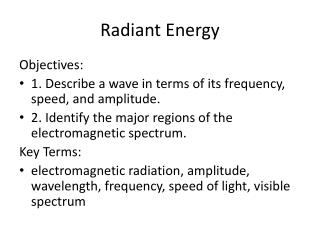DownloadDownload PresentationDownload Presentation- - - - - - - - - - - - - - - - - - - - - - - - - - - E N D - - - - - - - - - - - - - - - - - - - - - - - - - - -
##### Presentation Transcript

1. Radiant Energy Objectives: • 1. Describe a wave in terms of its frequency, speed, and amplitude. • 2. Identify the major regions of the electromagnetic spectrum. Key Terms: • electromagnetic radiation, amplitude, wavelength, frequency, speed of light, visible spectrum

2. Waves and Energy • A wave is a disturbance that transfers energy through a medium. • All waves can be characterized by the following four characteristics: amplitude, wavelength, frequency, and speed. • Light travels in electromagnetic waves. • Einstein called the quantum packets of energy photons. Each photon caries the exact amount of energy that the atom absorbed. • The Photon has a dual nature. (double transverse wave) • One part light and mass. • Or …one part electric and one part magnetic • This dual nature allows the photon to travel through space.

3. Amplitude • The maximum distance the molecules are displaced from the midline • Or... The distance from the midline to the crest or trough • As energy , amplitude  crest midline trough

4. Wavelength • The distance between 2 consecutive crests or troughs • Unit is the meter or nanometer (1nm = 1x10-9m)

5. Frequency • The number of crests of troughs passing a point per unit time • Transverse … crests(troughs)/second • Compression … compressions(rarefactions)/second • Unit is the hertz (Hz) 1Hz = 1/s or s-1

6. Speed of Waves • Speed in any given medium is constant. • Speed (c) = frequency (Hz)  wavelength () or c = Hz x  • If Hz  then or if Hz then  • A medium is any substance or region through which a wave is transmitted. • Solid, liquid, or gas • Fastest in solid & slowest in gas • Transfers energy only without any net movement • 2 properties of a medium affect wave speed • Density- in density without a change in medium slows the wave • Elasticity -  elasticity speeds up a wave

7. Light Energy • The atom is the source of all light. • When an atom absorbs a quantum of energy its electrons (e-) become excited and jump to higher energy orbits (excited state) • As the excited e- looses energy, it falls back to its original orbit (ground state) and releases a photon. • A photon is a tiny packet or bundle of energy that contains the exact amount of energy that the atom absorbed • The amount of energy a photon contains determines what type of light is emitted

8. Electromagnetic Spectrum • The frequencies of light created as an atom reaches quantum requirement is called a line spectrum. • a line spectrum (or emission spectrum)contains only certain frequencies of light which can be seen by passing the emitted light through a prism

9. Plank’s Equation • Plank's theory states that each element can only absorb a certain amount of energy (quantum) therefore it can only emit a limited amount of energy. Each element absorbs energy only when the quantum is reached. This was further explained by the equation: • Plank used this concept to describe why certain elements give of different color lights emit different colors. • E = hv if you know the amount of energy absorbed you can calculate the wavelength using the formula for wave speed (c = v x ) Frequency Hz Plank’s Constant 6.6262 x 10-34 Quantum energy requirement